Courses

# Definite integration, Business Mathematics & Statistics B Com Notes | EduRev

## B Com : Definite integration, Business Mathematics & Statistics B Com Notes | EduRev

The document Definite integration, Business Mathematics & Statistics B Com Notes | EduRev is a part of the B Com Course Business Mathematics and Statistics.
All you need of B Com at this link: B Com

## Definite Integrals

Integration can be used to find areas, volumes, central points and many useful things. But it is often used to find the area under the graph of a function like this: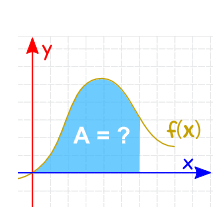The area can be found by adding slices that approach zero in width:

And there are Rules of Integration that help us get the answer.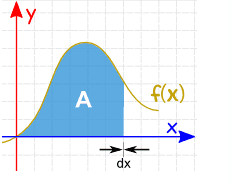## Notation

he symbol for "Integral" is a stylish "S"
(for "Sum", the idea of summing slices):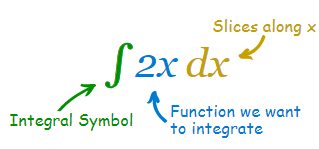After the Integral Symbol we put the function we want to find the integral of (called the Integrand),

and then finish with dx to mean the slices go in the x direction (and approach zero in width).

## Definite Integral

Definite Integral has start and end values: in other words there is an interval (a to b).

The values are put at the bottom and top of the "S", like this: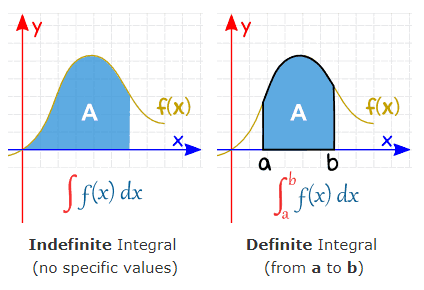We can find the Definite Integral by calculating the Indefinite Integral at points a and b, then subtracting:

### Example:

The Definite Integral, from 1 to 2, of 2x dx: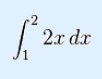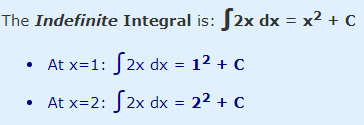Subtract:

(22 + C) − (12 + C)

22 + C − 12 − C

4 − 1 + C − C = 3

And "C" gets cancelled out ... so with Definite Integrals we can ignore C.

In fact we can give the answer directly like this: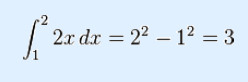Check: with such a simple shape, let's also try calculating the area by geometry: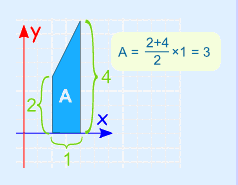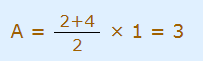Yes, it does have an area of 3.

(Yay!)

Let's try another example:

### Example:

The Definite Integral, from 0.5 to 1.0, of cos(x) dx: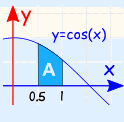(Note: x must be in radians)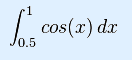The Indefinite Integral is: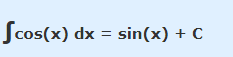We can ignore C when we do the subtraction (as we saw above):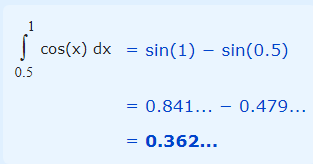And another example to make an important point:

### Example:

The Definite Integral, from 1 to 3, of cos(x) dx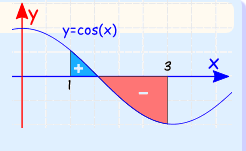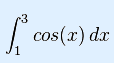Notice that some of it is positive, and some negative.
The definite integral will work out the net area.

The Indefinite Integral is:cos(x) dx = sin(x) + C

So let us do the calculations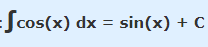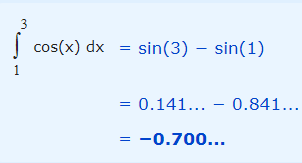So there is more negative than positive parts, with the net result of −0.700....

### Example: What is the area between y = cos(x) and the x-axis from x = 1 to x = 3?

This is like the example we just did, but all area is positive (imagine you had to paint it).

So now we have to do the parts separately:

• One for the area above the x-axis
• One for the area below the x-axis

The curve crosses the x-axis at x = π/2 so we have:

From 1 to π/2: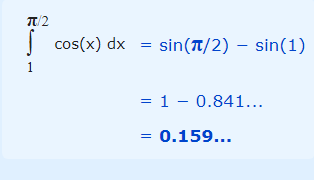From π/2 to 3: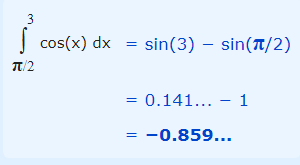That last one comes out negative, but we want it to be positive, so:

Total area = 0.159... + 0.859... = 1.018...

This is very different from the answer in the previous example.

## Continuous

Oh yes, the function we are integrating must be Continuous between a and b: no holes, jumps or vertical asymptotes (where the function heads up/down towards infinity).

### Example:

A vertical asymptote between a and b affects the definite integral.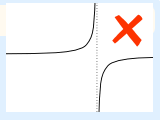## Properties

### Reversing the interval

Reversing the direction of the interval gives the negative of the original direction.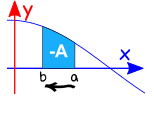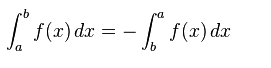### Interval of zero length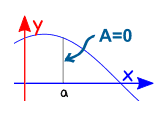When the interval starts and ends at the same place, the result is zero: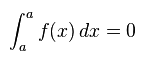### Adding intervals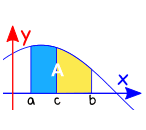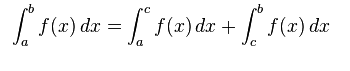## SummaryThe Definite Integral between a and b is the Indefinite Integral at b minus the Indefinite Integral ata.

Offer running on EduRev: Apply code STAYHOME200 to get INR 200 off on our premium plan EduRev Infinity!

122 videos|142 docs

,

,

,

,

,

,

,

,

,

,

,

,

,

,

,

,

,

,

,

,

,

,

,

,

;